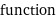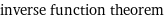# GET TUTORING NEAR ME!(800) 434-2582

/ Get Math Help

## One-to-one Function

Alternate name
Definition

Let f(x) be a function. If f(x) never takes on the same value twice, then f(x) is a one-to-one function. More explicitly, f(x) is one-to-one if f(x_1) = f(x_2) implies x_1 = x_2.

Details